This page usesand(click images to validate).
You will need a standards-compliant browser to view it properly. Mozilla 1.x and Opera 6.x are known to work if your default font contains all mathematical symbols specified by HTML. Internet Explorer seems to have trouble with some mathematical symbols even if the font contains them.

# Visualization of Natural Deduction as a Game of Dominoes

## Abstract

Diagrammatic reasoning systems that allow conducting a proof within a certain logic have existed for some time, especially as teaching tools. However, few systems exist that work without any textual formulae. The domino system presented here is a purely graphical notation for natural deduction. A formula is represented as a pattern on a domino tile and a simple domino game is used as visualisation of a natural deduction proof. The game has been implemented as a Java program that acts as an interactive theorem prover for classical propositional logic. The program demonstrates that graphical reasoning systems have some advantages but also disadvantages. In conjunction with the domino project, a tautology generator, an interactive theorem prover in text mode and a program that automatically constructs a natural deduction proof for a given tautology have been developed.

## 1 Introduction

### 1.1 Reasoning and Diagrams

There are different ways of reasoning. The natural way for most people is reasoning on the semantic level. Even mathematicians, although they use precise definitions and a very formalized way of presentation, generally conduct proofs using semantic arguments. Diagrams have always played an important role in this reasoning style.

Another way of reasoning, practiced in its pure form almost exclusively by logicians, is to work on the syntactic level, using a formal and abstract language and exact rules governing the creation and transformation of statements in this language. The use of diagrams in syntactic reasoning has not found many followers. One important reason for this is probably that drawing and manipulating large numbers of diagrams is tedious and inefficient, as is the typesetting of the results of this process. However, modern computer technology can help with both issues.

The idea of syntactic reasoning based on diagrams is anything but new. At the end of the 19th century, when modern mathematics was still in its infancy, C. S. Peirce suggested a formal deduction system he called "Existential Graphs" that is based on diagrams (for an introduction to this system see ) and is as powerful as first-order logic. Other reasoning systems based on diagrams have been developed, usually with a more specific area of application, e.g. a system for reasoning with spider diagrams (see ) .

There are many computer programs that involve diagrammatic reasoning, usually for teaching purposes. Most of these programs, like for instance the Hyperproof system (see ) , use diagrams only to support other reasoning styles. The diagrams employed by these programs and in fact even the diagrams involved in most reasoning systems that are syntactic, such as the spider diagrams mentioned above, usually have a strong semantic element and an intuitive reading.

The program described in this paper is different. Although it does not implement Peirce's Existential Graphs, it is based on the same idea of conducting a proof without leaving the diagrammatic level. Working with such a system is radically different from how proofs are usually developed. Peirce once wrote:

By diagrammatic reasoning, I mean reasoning which constructs a diagram according to a precept expressed in general terms, performs experiments upon this diagram, notes their results, assures itself that similar experiments performed upon any diagram constructed according to the same precept would have same results, and expresses this in general terms. This was a discovery of no little importance, showing, as it does, that all knowledge without exception comes from observation.

So, according to Peirce, the heart of diagrammatic reasoning is experimentation with diagrams on the syntactic level to gain new insights based on observation. This is much unlike the common use of diagrams to visualize things already known. Getting used to this different working style is difficult at first as it requires much "unlearning". It is not possible to work productively with an abstract diagrammatic system if you keep translating between the diagrams and another system, such as first-order logic. The reader should keep this in mind, when working with the Domino system detailed below.

### 1.2 Natural Deduction

Unlike Peirce's Existential Graphs, the Domino system described below is not an all-new logical formalism. It is based on the system of natural deduction presented by G. Gentzen in . While natural deduction is a system for the whole of first-order logic, the Domino system is restricted to propositional logic. To simplify things even further, only classical logic is used, which requires only 3 inference rules. The following paragraphs will introduce natural deduction regarding classical propositional logic. It is assumed that the reader already has some familiarity with the matter, so the overview will be brief, just enough to serve as a reminder and to establish the terminology and syntax that will be used when describing the Domino system.

#### 1.2.1 Formulae, → and ⊥

A formula may contain bound propositional variables denoted by strings of capital letters (A, B, C,..., AA, AB,...), unbound propositional variables denoted by a capital X followed by a non-negative integer (X0, X1, X2,...), the binary operator and the symbol . Parentheses are used for grouping.

The symbol (read "false" or "bottom") is a formula that is always false.

The operator (read "arrow" or "implies") is the implication operator. An implication is false if the formula on the left side (the premise) is true and the formula on the right side (the conclusion) is false. In all other cases the implication is true. The implication ⊥→B is true, for instance.

Bound propositional variables stand for statements that can be either true or false, such as "The sun is shining.".

Unbound propositional variables are meta-variables that stand for formulae. X0 could be a placeholder for the formula AB, for example.

The symbols and are sufficient for classical propositional logic. Other commonly used operators can be seen as abbreviations for formulae using these two symbols:

• ¬A   ("not A")    = A→⊥
• AB("A or B")   = ¬AB
• AB("A and B")  = ¬ (¬A∨¬B)

A special type of formula is the tautology. A tautology is a formula that is always true regardless of what the propositional variables stand for. E.g. the formula AA is a tautology, because it is true regardless of whether A is true or false.

#### 1.2.2 Assumptions and Derivations

Natural deduction (in the limited form presented here) is a calculus for syntactically deriving tautologies in propositional logic. The system is correct and complete, i.e. if a certain formula is a propositional logic tautology, it can be derived by natural deduction and if a formula can be derived by natural deduction, this formula is a tautology with respect to propositional logic. This means that a derivation of a formula in the system of natural deduction is a proof of that formula and the term proof will be used as a synonym for derivation in this article.

A natural deduction proof is usually presented in a tree-like notation where the derived formula is the root, usually written at the bottom. The leaves of the tree are formulae referred to as assumptions. The inner nodes of the tree correspond to applications of the derivation rules detailed further below. Each rule is written as a horizontal line with exactly one formula (the conclusion) below that line and one or more formulae (the premises) above that line. In addition to this, some rules are written with one or more formulae in square brackets to the right of the line. These are assumptions that this rule discharges. In order to be a valid derivation for a tautology, all of the assumptions found in leaves of the tree must be discharged (noted by being put in square brackets). An assumption is discharged if one of its ancestors in the tree is a rule that discharges it. The following is an example of a valid derivation:

[(A→⊥)→⊥]   [A→⊥]

[A→⊥]
A
[(A→⊥)→⊥]
((A→⊥)→⊥)→A

#### 1.2.3 Rules of Inference

To get a complete (and correct) calculus for classical propositional logic, the following three rules (more properly rule schemata) of inference are sufficient. All other rules can be derived using just these three.

X1
[X0]
X0X1

introduction

X0    X0X1

X1

elimination/modus ponens

[X0→⊥]
X0

## 2 The Domino Metaphor

### 2.1 Introduction

Dominoes is a game played with rectangular tiles. Each tile is divided in two squares that are labelled with number symbols like the faces of a standard dice. There are many games that can be played with domino tiles. One of the most simple variants of the Dominoes game begins with a single tile and each player having a pile of tiles. A player may play one of his tiles by connecting it to an unconnected end of a tile played earlier. The two connected squares must have matching numbers.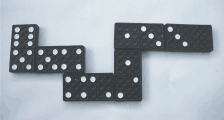Natural deduction follows similar principles. It is very common to begin with the tautology to prove and to apply inference rules backwards until only discharged assumptions remain. When seen as a domino game, each inference rule corresponds to a domino tile, with premise and conclusion corresponding to the labels on the two squares of the tile. The main differences to a real game of dominoes are

• Inference rules are directed. A premise can only be connected to a conclusion and vice versa.
• The modus ponens inference rule has two premises and consequently three connection points.

Aside from these differences the domino game models natural deduction rather well. The number of different square labels needs to be higher than used for a real domino game, of course. Although arbitrary symbols could be chosen, it is desirable to have a systematic mapping from formulae to square labels. The picture below demonstrates a possible rendering of a proof for ⊥→(A→(B→(CD))) as a game of dominoes. The proof does not use modus ponens, so there are no branches. Note that discharged assumptions are not shown in the picture.### 2.2 Mapping Derivations to Dominoes

The following paragraphs give a detailed description of the mapping between natural deduction derivations and the dominoes game as it has been implemented. As mentioned before, the domino system described here is not a new logical formalism. It is simply a different notation for natural deduction, used to create a special kind of Graphical User Interface (GUI) for an interactive theorem prover. Consequently, the mapping described here is a straightforward 1-to-1 mapping, so that the correctness and completeness results for natural deduction apply unchanged.

#### 2.2.1 Mapping Formulae to Color Patterns

The labels used on the domino tiles correspond to the premises and conclusions of the inference rules. While it would be possible to use a formula directly in its textual form to label a square of a tile, this is not desirable in practice. The space available on a domino tile is very limited, so the formula would have to be printed in a very small font when presenting the tile on screen. Furthermore, as the domino notation is intended only for use in a GUI, it is only reasonable to make full use of the graphical nature of the environment by presenting formulae in a way that offers better visual clues than traditional text notations. Last but not least, a colorful presentation makes the program overall more enjoyable.

For the purpose of the following definition, let pattern(X0) denote the rectangular pattern assigned to the formula X0. pattern(X0) is defined as follows:

Case  X0=⊥ :
The pattern for is a solid red rectangle framed by a black and a white border:Case  X0=A, X0=B, ... :
Every propositional variable is assigned a unique color that is not white, black or red. The pattern for a propositional variable is a solid rectangle of the variable's assigned color, framed by a black and a white border.
Examples: pattern(A) =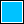, pattern(B) =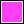Case  X0=(X1X2) :
The pattern for an implication is a rectangle R that is split in two rectangles R1 and R2. The pattern of R1 is pattern(X1) and the pattern of R2 is pattern(X2). The split is done according to the following rules:
• If R is not the result of a split, i.e. if X0 is not part of another implication, the split is a horizontal split, with R1 as the top and R2 as the bottom rectangle.
• If R is the result of a split, i.e. if X0 is an operand of an implication for which the pattern is being computed, the split axis for R is perpendicular to that of its parent rectangle. If R is split horizontally, then R1 is the top rectangle and R2 is the bottom rectangle. If R is split vertically, R1 is the left and R2 is the right rectangle.
Examples: pattern(AB) =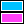, pattern((AB)→C) =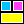, pattern(A→(BC)) =, pattern(((AB)→C)→D) =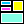, pattern((AB)→(CD)) =It is obvious that with the alternating split axis and the rule that the first split must be horizontal, it is always possible to unambiguously determine the exact sequence of splits that lead to any given pattern. The black and white borders around the rectangles make sure that two adjacent rectangles can never appear as a single, larger rectangle, even if they have the same color. As a consequence, the mapping from formula to pattern is easily reversed.

#### 2.2.2 Mapping Inference Rules to Tiles

Now that each formula has been assigned a color pattern, mapping inference rules to domino tiles is trivial. A rule is characterised by its premises, its conclusion and the assumptions it discharges. All of these are formulae. Each formula X0 is represented by a block that has pattern(X0) on its top. All of these blocks are connected to form the tile that represents the inference rule.

One important thing has to be taken into account. Conclusion, premises and discharged assumptions play different roles and therefore need to be distinguished. The representation presented here distinguishes them by the color of the sides of the blocks. The conclusion of a derivation rule is represented by a block with red sides, the premises are represented by blocks with green sides and the discharged assumptions are represented by blocks with blue sides. These blocks will be referred to as red blocks, green blocks and blue blocks respectively.

The arrangement of the blocks is not important as long as the blocks that represent conclusion and premises are not completely surrounded by other blocks, which would make the connection of another tile impossible. Because keeping track of the discharged assumptions is very important, the respective blocks are presented as being stacked on top of another block, which makes them stick out visually. The following pictures show examples of instances of the three inference rules of natural deduction:

A
[B]
BA

is represented asAB  (AB)→C

C

is represented as[C→⊥]
C

is represented as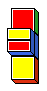#### 2.2.3 The Rules of the Game

The rules of the game are easy. The game begins with a single tile that has a single green block that represents the tautology to be proven and no red block. This would correspond to a derivation rule with just a premise and no conclusion. Every turn, a tile representing a derivation rule is connected to a tile placed earlier. The red block of the newly placed tile must be connected to exactly one green block of the other tile. Two green blocks can not be connected. The usual domino rule applies that the two blocks being connected must show the same pattern.

In a real domino game, players only have a limited number of tiles. In natural deduction domino, all tiles corresponding to instances of the three derivation rules are available without limitations at all times.

The structure built up during the course of the game is a tree with the start tile as root and one or more green blocks as leaves. This tree will be referred to as the domino tree. A leaf of the domino tree is said to be closed if the green block that marks this leaf shows the same pattern as at least one blue block of the tile the leaf belongs to or a tile that is an ancestor of this tile in the domino tree. If all leaves are closed, the domino tree is said to be closed. Otherwise it is open. The following pictures show some closed and some open domino trees. To emphasize closed leaves, blue blocks have been connected to them. This is just a matter of presentation and not an actual part of the mapping between proof and domino game.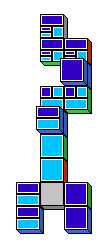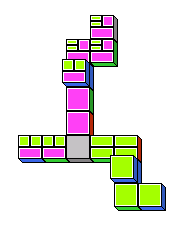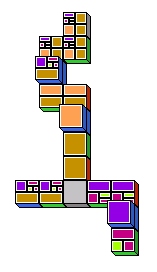The game is finished when all leaves of the domino tree are closed. Rules for scoring and determining which player has won the game are not provided here because they are not relevant for the logical mapping between domino game and natural deduction. It is easy to see that any closed domino tree for a game finished according to the above rules corresponds to a valid derivation of a tautology. Likewise, every correct derivation can be transformed into a domino tree. Domino trees are just a different notation for natural deduction derivations.

## 3 The Domino Program

### 3.1 Program Description

The domino metaphor for natural deduction has been implemented in Java. The program is available at . It can be downloaded for local use or played on-line with Java-enabled browsers. Full source code is included.

The program has one main window that displays the domino tree for the current game. Domino tiles are shown in an isometric perspective. The blocks are labelled with color patterns as have been described, with a small extension. Because the number of visually distinct colors is limited, multi-letter propositional variables are not represented by a single-color solid rectangle. Instead they are represented by concentric rectangles, each of which represents a single-letter. This extended mapping is still unique and reversible.

The program is controlled by the mouse or alternatively by a combination of mouse and keyboard. The user requests new domino tiles and places them in the main window according to the rules that have been explained.The program offers some useful features beyond the actual domino game. The perhaps most important feature is the ability to create a text representation in standard notation of the proof corresponding to the current domino tree. This allows a direct comparison between the two notations.Another interesting feature is the ability to create custom tiles as shortcuts for combinations of several tiles. Saving and loading these custom tiles is supported. The format used for the tile files is an easy to read and edit text format which allows the creation of tiles that go beyond classical logic and the rules described in this paper. It would be possible to restrict the game to intuitionistic logic, for example.

The program implements the mapping between natural deduction and dominoes with some differences compared to the description given in this paper. The first difference is the terminology used in the manual and the menus. Terms such as "modus ponens" and "tautology" are avoided. Instead non-technical names are used that are more appropriate for a computer game. The intention is to increase the appeal of the program to people without a scientific background. This is desirable because one of the goals of this project has been to create an environment that even persons without any background in logic should be able to use. Although no further research in this direction has been done as part of this project, studying the way the domino program is used by people without background knowledge could yield interesting results.

Another difference is that by default the start tile presented by the program does not correspond to the rules of the game as they have been presented in this article. Because it is so common to begin the proof by applying the introduction rule to the tautology to be proven in order to simplify it and discharge assumptions at the same time, the game automatically applies the  introduction rule as often as is possible, thereby simplifying the pattern on the start tile's green block while at the same time providing some blue blocks. This does not affect correctness or completeness and the feature can be turned off in the Level menu.

The most important difference between the domino metaphor as discussed in this paper and the actual implementation concerns the tiles corresponding to inference rules. To play the game, all tiles corresponding to instances of the derivation rules must be available to a player. However, there is an infinite number of such instances, which means that it is unfeasible to provide the tiles explicitly. One possible way to solve this problem would be to have the user specify the premises and conclusions a rule is to be instantiated with. This would either require the entering of formulae which would undermine the whole concept of using a purely visual representation, or it would require some way to specify the patterns directly, which would likely be as inconvenient.

The solution implemented in the Domino program is different. It could be described as indirect or lazy instantiation. It is based on the observation that an inference rule does not need to be instantiated right away. This is best explained with an example. Let's assume the user wants to derive A→(BA) (i.e. the corresponding tile is the start tile). As a first step he wants to attach a tile that corresponds to the following inference rule:

BA
[A]
A→(BA)

This rule is an instance of the introduction rule (schema):

X1
[X0]
X0X1

When the user requests an introduction tile, a dialog box could be presented, asking him to enter formulae for X0 and X1 and the user would enter A and BA respectively. The tile would be created and the user could attach it to the A→(BA) block of the start tile. However, it turns out that requiring the user to enter bindings for X0 and X1 directly is unnecessary. The domino rules say that two tiles can only be connected if the adjacent squares show the same pattern. If the user wants to attach an introduction tile to an A→(BA) block, there is no choice for X0 and X1. If they are not A and AB respectively, the tiles cannot be connected. To take advantage of this observation, when the user requests an introduction tile, a meta-tile is created that corresponds to the schema without being an instance. For the purpose of constructing the patterns for the blocks of this tile the unbound variables X0 and X1 in the schema formulae are treated as if they were bound variables distinct from all other bound variables. The following is an example of such a meta-tile: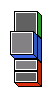Here, shades of gray are used in the patterns as colors for the unbound variables. This doesn't create a conflict because the program does not use shades of gray for bound propositional variables. When this meta-tile is connected to another tile, the gray rectangles are replaced with the corresponding patterns on the block the tile is connected to. In other words, the tile is instantiated indirectly through the connection made.

This indirect instantiation has an interesting effect when modus ponens tiles are used. As you can see in the following picture, attaching a modus ponens tile to another tile does not bind all of its wildcard patterns.This is easy to understand when you look at the modus ponens inference rule:

X0    X0X1

X1

The variable X0 is not present in the conclusion so when the red block that corresponds to the conclusion is connected to a green block, the variable X0 does not matter at all. To bind this variable, too, another tile is required. In the following picture, a blue block is attached to close a leaf. This binds X0.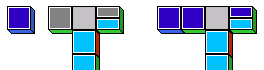It is possible to connect other meta-tiles to the branches of a modus ponens tile, as demonstrated in the following picture. This does not bind the wildcard patterns, but it merges the structural information they provide. In this case one wildcard pattern has a split and the other has not. The split pattern is more specific than the unsplit pattern, because the corresponding formula must have at least one , whereas the unsplit pattern can represent any formula whatsoever. The domino rule mandates that both blocks must show the same pattern. The information contained in the split pattern that it stands for an implication must not be lost obviously, so the unsplit pattern changes to match the split pattern.It is important to understand that the introduction of meta-tiles does not in any way extend or change the underlying logic. Each wildcard pattern on a meta-tile is just a placeholder for a pattern representing a formula that contains no unbound variables. This applies even if a finished domino tree still contains wildcard patterns like the following: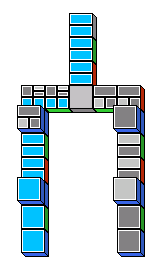Because the inference rule schemata make no restrictions regarding instantiation of unbound variables, the proof corresponding to the above tree is valid, regardless of the formulae bound to the remaining unbound variables. So, in order to eliminate the remaining unbound propositional variables, it is permissible to replace them all with the bound variable A, for instance.

### 3.2 Implementation Overview

The following sections will take a look at some aspects of the program's implementation that concern the internal representation of formulae and domino trees. For a comprehensive and in-depth look at the implementation, readers are referred to the source code that is provided with the program and the javadoc documentation that can be generated from it.

The source code is split across three packages: domino.core, domino.ui and domino.logic. The classes that will be discussed here all belong to the domino.logic package. This package can be divided into three layers. The first layer is the formula layer, provided by the FormulaSyntaxNet class that is used for the low-level representation of formulae. Building on top of and very closely connected to this layer is the domino tree layer, provided by the FormulaDominoTree class. Above this layer is the application layer formed by the classes/programs that make use of FormulaDominoTrees as representations of natural deduction derivations.

### 3.3 The FormulaSyntaxNet Class

One question that stood at the beginning of the development of the domino program was that of how domino trees should be represented internally. This question inevitably lead to the question of how formulae should be stored and manipulated. Although mathematically harmless, the desire to implement the indirect instantiation feature in the program's user interface turned out to be the dominating factor when designing the data structures dealing with formulae. Most of the complexity but also the flexibility of the FormulaSyntaxNet and FormulaDominoTree classes is a direct consequence of design choices made to add support for this feature.

In order to support indirect instantiation, it has to be possible to unify two or more formulae. Unification identifies the unbound propositional variables in one formula with parts of another formula and vice versa, with the goal of making the two formulae equivalent. If the formula A→(BC) is unified with the formula X0X1 for instance, X0 is bound to A and X1 is bound to (BC). With this binding, the second formula is equivalent to the first. A slightly more complicated example is the unification of X0→(BC) with AX1 that results in the same bindings for X0 and X1.

Not all formulae can be unified. The unification of AB and X0C is not possible because B and C are different bound propositional variables that can not be identified with each other. The unification of A→(X0X1) with AB is also not possible, because no binding of X0 and X1 can make X0X1 equivalent to B.

Looking at the simple examples above, unification seems to be easy to implement using a table that maps unbound variables to the formulae they are bound to. Unfortunately, things are not as easy as the simple examples suggest. One issue that has to be dealt with is the possibility that a unification occurs between two unbound variables as happens when unifying X0X1 with X2X3. This unification leaves all four variables unbound. It does, however, establish a connection between X0 and X2 and a connection between X1 and X3. Suppose that, after this unification, another unification takes place, unifying X0X1 with AB, this unification must bind not only X0 and X1, but also X2 and X3. A series of unifications of formulae containing many unbound propositional variables can quickly lead to a complex web of connections.

Even with the complications arising from unifying unbound variables with each other, a simple table-based approach seems possible. Unbound variables that have been unified could be made to point to a common entry in the table and successive unifications would update the respective entries and pointers. This method is simple to implement and works well if unifications are final. Applied to the domino metaphor, unifications being final corresponds to a rule that says that a domino tile can not be removed once it has been placed. Indeed, such a rule exists in real domino games, but with such a rule it would be possible for the user to find himself in a dead end during the game. So it is essential to give the user the ability to remove tiles from a domino tree. Unfortunately, providing this feature makes the implementation more difficult. An easy way to implement the feature would be to store a history of unifications, similar to the undo information in a text editor. This approach would limit the user to removing tiles in the order they were placed. But users will often want to remove tiles from different leaves of the domino tree and would perceive the restrictions imposed on their ability to do so as arbitrary and counter-intuitive.

To address all of the issues mentioned above, the data structure for managing formulae and unifications needs to be more complex than a simple table that maps unbound variables to their bindings. The data structure that was chosen for the domino program and implemented in the FormulaSyntaxNet class is a net of interconnected trees. The following paragraphs will take a look at this structure.

As long as no unifications are involved, a FormulaSyntaxNet is just a kind of syntax tree. This tree can have three types of node. The first category is made up by bound leaf nodes. A bound leaf is a leaf that is labelled with the name of a bound propositional variable as depicted in the following picture:The second node type is the unbound leaf node. An unbound leaf is a leaf node that does not have a label.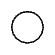The third category of nodes are the branch nodes. In a syntax tree a branch node corresponds to an operator, that has its operands as branches. Because is the only operator that can occur in the formulae used here, all branch nodes are binary nodes whose left branch is the premise of an implication and whose right branch is the conclusion.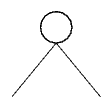With branch nodes, unbound and bound leaves, all the formulae needed for classical propositional logic can be represented. The following pictures show the FormulaSyntaxNets for (BX0)→X1, X2→(AC) and (AX3)→X4 respectively. Notice that the variable names used for unbound variables in the formulae are arbitrary as long as the same unbound variable has the same name whenever it occurs and two different unbound variables have different names. In the tree structure, names are not necessary for unbound nodes. If the same unbound variable occurs multiple times in a formula, the corresponding FormulaSyntaxNet contains only one unbound node for that variable. This can lead to deformed trees where multiple different branches have the same leaf.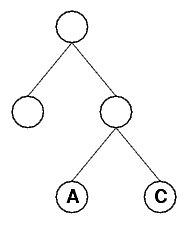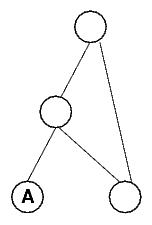When dealing with FormulaSyntaxNets, you should keep in mind that while every formula has a corresponding FormulaSyntaxNet, not every FormulaSyntaxNet corresponds to a formula. A FormulaSyntaxNet can be recursive as is demonstrated in the following picture. This FormulaSyntaxNet would correspond to an infinite formula, but such formulae are not permitted here.Now that the representation of basic formulae as FormulaSyntaxNets has been described, unification will be introduced. For this purpose, a fourth node type, the forward node, is necessary. Forward nodes are an extension of unbound leaves. A forward node carries a reference to every node that is equivalent to it because of unifications. So, an unbound leaf is a forward node that has not been unified with any other node. Notice, that a forward node may refer to other forward nodes and these refer back to it, because equivalence is symmetrical.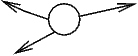The name forward node refers to the way most algorithms working on FormulaSyntaxNets treat them. A forward node that has at least one reference (i.e. a forward node that is not an unbound leaf) is processed by picking one of the nodes it refers to and processing that node. In other words, the processing is forwarded to one of the referenced nodes. Because all nodes referred to by a forward node are equivalent, it does not matter which one is picked for processing. This is only a simplified description, of course. In practice it is at least necessary to take precautions to prevent endless loops, because the node being picked could itself be a forward node, and as such would have a reference back to the original forward node which must not be picked again.

Forward nodes are only created when unifying FormulaSyntaxNets. The following pseudo-code gives an overview of the unification algorithm. In this pseudo-code, as well as the actual Java implementation, a variable of type FormulaSyntaxNet refers to a single node within the net.

```unify(FormulaSyntaxNet a, FormulaSyntaxNet b)
{
if (a is the same node as b OR
a and b have already been unified) return

if (a is bound leaf AND b is bound leaf)
{
if (label of a != label of b)
throw UnificationNotPossibleException
}
else
if (a is branch node AND b is branch node)
{
unify(left branch of a, left branch of b)
unify(right branch of a, right branch of b)
}
else
if (a is unbound or forward node OR b is unbound or forward node)
{
let x be the first unbound or forward node in the list (a,b)
let y be the other node

if (y is bound node or branch)
{
add a reference to y to x's list of references

for every node p from x's list of references
{
unify(p,y)
}
}
else if (y is unbound or forward node)
{
add a reference to y to x's list of references
add a reference to x to y's list of references

for every pair of nodes (p,q)
where p is from x's list of references
and   q is from y's list if references
{
unify(p,q)
}
}
}
else throw UnificationNotPossibleException
}
```

The following picture shows the result of unifying the formula A→(BX0) with the formula X1→(BX2).There are some things that the above algorithm does not show but that are important nevertheless. One thing that is missing above is code to remove all newly created references if the unification fails, to restore the nets a and b to their original states. Another omission is code to recognize unifications that would cause the creation of a recursive FormulaSyntaxNet. As has been mentioned, a recursive FormulaSyntaxNet corresponds to an infinite formula which is not allowed. Readers interested in how these details are handled in the Domino program should consult the source code.

The FormulaSyntaxNet class provides the functionality needed to work with formulae connected through unifications no matter how complex. A FormulaSyntaxNet can even represent an infinite formula. It is obvious that for implementing the domino metaphor all this flexibility is mostly unnecessary. Furthermore, FormulaSyntaxNets with all their pointers and their complex interconnections are difficult to work with and a real nightmare to debug.

To address these issues, the FormulaDominoTree class has been introduced as a layer above FormulaSyntaxNet. FormulaDominoTrees provide a higher level of abstraction because they work on the level of domino tiles and domino trees. This serves two purposes. First, it reduces the amount of application layer code, because one operation on a FormulaDominoTree may perform many operations on the underlying FormulaSyntaxNet(s). The second and more important purpose is to enforce the rules of natural deduction. The FormulaDominoTree class is the implementation of the domino tree as it was introduced earlier, and as such directly corresponds to natural deduction. It is possible to automatically convert any FormulaDominoTree to the standard textual notation for derivations. The ProofFormatter class implements this conversion.

A single instance of the FormulaDominoTree class represents a derivation rule. It has one FormulaSyntaxNet that represents the conclusion, one for each premise and one for each discharged assumption. These FormulaSyntaxNets are not separate. Whenever possible they physically share nodes, so that within an atomic FormulaDominoTree instance, unifications are not necessary. Consequentially, an isolated FormulaDominoTree does not have any forward nodes in its FormulaSyntaxNets.

In addition to the FormulaSyntaxNets, a FormulaDominoTree holds references to other FormulaDominoTrees. There is one reference for the conclusion and one for each premise. These references are used to connect atomic FormulaDominoTree instances to form a tree, just like domino tiles are connected to form the domino tree. Whenever the conclusion connection of one FormulaDominoTree is connected to a matching premise connection of another FormulaDominoTree, the respective references are updated and the corresponding FormulaSyntaxNets are unified. This directly corresponds to the connecting of domino tiles in the domino program to build the domino tree. Notice that, although technically FormulaDominoTree is the class of a single node of the tree, the name will also be used to refer to the tree that is rooted at that node.

The domino game uses three types of atomic FormulaDominoTrees that correspond to the three types of domino tiles in the domino metaphor, which in turn correspond to the three inference rules presented for classical propositional logic. The following pictures illustrate these three types. In the pictures, the node that corresponds to the conclusion of the rule has a double border, numbers mark the unbound leaf nodes corresponding to the premises and the letter "d" marks the FormulaSyntaxNet representing a discharged assumption.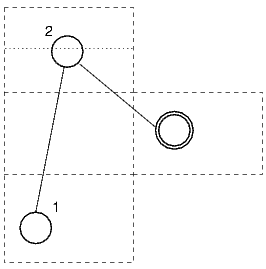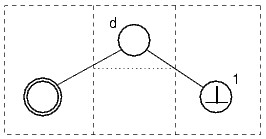There is a fourth type of FormulaDominoTree that is used to close a leaf of the domino tree just like the blue blocks are used in the domino program. This special FormulaDominoTree has only one FormulaSyntaxNet that is unified with a discharged assumption of an ancestor FormulaDominoTree. With these four atomic FormulaDominoTrees, any domino tree can be represented.

The following sequence of pictures shows how the FormulaDominoTree is built up during the first steps of a proof for the formula A→((AB)→B), using the diagram notation described above.When the FormulaSyntaxNet class was introduced, the problem of removing tiles from a domino tree was already mentioned. With the FormulaDominoTree class this problem is now easy to solve. Whenever a subtree of a FormulaDominoTree is removed, all that has to be done is to undo unifications with FormulaSyntaxNets belonging to the subtree being removed. The algorithm implemented to do this is very simple. It removes all forward node connections from all FormulaSyntaxNets and after removing the subtree reunifies those corresponding to premise/conclusion connections that remain. This algorithm works because atomic FormulaDominoTrees never contain forward nodes since their FormulaSyntaxNets physically share nodes. All forward node connections in all FormulaSyntaxNets within a FormulaDominoTree are the result of connecting atomic FormulaDominoTrees, and as a consequence they can all be reconstructed from the premise/conclusion connections.

The above solution is obviously not very efficient. In some special cases, the program uses a more efficient algorithm that works by assigning every FormulaSyntaxNet a reference to the FormulaDominoTree that contains it. Using these owner references it is easy to limit the removal of connections to those FormulaSyntaxNet nodes that belong to the domino tile being removed. However, one issue makes this method unfit for general use. The problem is illustrated in the following picture sequence (for clarity's sake only three FormulaSyntaxNet nodes are shown instead of three complete FormulaDominoTrees).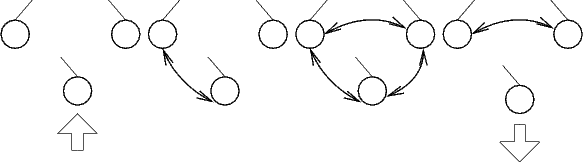The illustration demonstrates that when an unbound leaf X0 is unified with another unbound leaf X1 and then with yet another unbound leaf X2, this creates a cross-connection between X1 and X2. This happens because forward nodes by definition have references to all nodes that are equivalent (through unification), and equivalence is transitive. The cross-connection remains even after removing X0, because it is a connection between nodes that belong to different FormulaDominoTrees than X0, so that the owner-based algorithm does not touch it. While the domino tree remains logically valid, this effect is confusing for the user and might in some cases even result in a tree that can not be closed anymore. This issue can only arise when the same discharged assumption is used to close two different leaves. To eliminate the problem, the cross-unifications in question would have to be tracked, but this is currently not implemented because the trees as they occur when using the program are so small that the performance loss of the complete rebuilding algorithm has no practical significance.

During the development of the main program, a few other interesting programs were written that make use of the FormulaDominoTree class. They are all similar in the respect that they construct FormulaDominoTrees by repeatedly connecting copies of the three basic FormulaDominoTrees that correspond to the three rules of inference. In addition to this, discharged assumption FormulaDominoTrees are used to close leaves. This process is repeated until a tree has been generated with no open leaves remaining. This tree is converted to an ASCII representation of the corresponding proof in standard notation using the ProofFormatter class and then written to standard output.

#### 3.5.1 TautologyGenerator

This class provides a method for generating a propositional logic tautology with a proof in the form of a FormulaDominoTree. When used as a stand-alone program, the tautology is output as an infix formula together with a proof in standard notation. Parameters can be passed to influence the relative frequency with which the different inference rules are used in the proof. Note, that the proof is by no means a minimal proof. The program will often generate complex proofs for trivial tautologies. The domino.core.LevelGenerator is a slightly modified version of this class that aims to produce more complex tautologies to be used as levels in the domino game.#### 3.5.2 TextModeProver

In a way this class can be seen as the ancestor of the domino program. It offers a simple text mode interface for proving a tautology. The program begins with the tautology to prove and enumerates all possibilities for applying an inference rule, presenting the new premises that have to be proven if a rule is applied and the assumptions the rule discharges. The user simply enters a number to select one of the presented possibilities and the respective rule is applied. This continues until the FormulaDominoTree that is built up in this manner is closed. Finally the constructed proof is output in standard notation.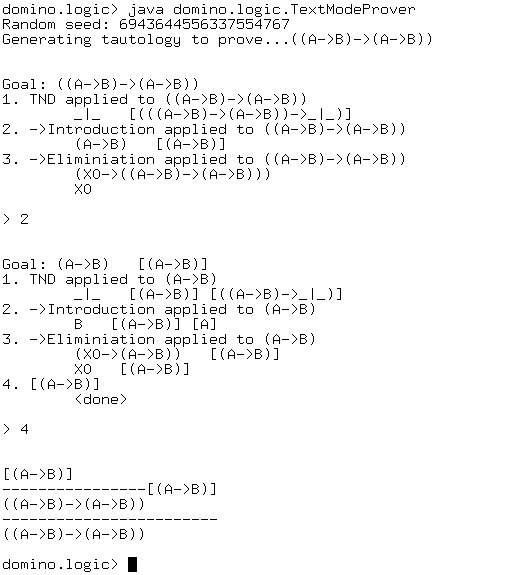#### 3.5.3 Prove

This is a particularly interesting class. It works as a stand-alone program that takes a tautology as input and finds a proof for it. The program does this by constructing FormulaDominoTrees beginning with the tautology to be proven, until a closed FormulaDominoTree has been found. Because breadth-first search consumes too much time and resources, the program uses heuristics to keep the number of trees low and to focus on those trees that are more likely to lead to a fast conclusion of the search. Because of these heuristics, the constructed proof is not minimal, but this is compensated by a proof-simplification step that is usually capable of reducing the proof to a minimal one.

No attempt was made to ensure that the heuristics employed maintain the completeness of the breadth-first search, i.e. it is well possible that a tautology exists for which the program can not find a proof because the heuristics discard all trees that would prove it. However, with the several hundred tautologies tested with this program this problem has not been observed.

It needs to be said that Prove was not written to be a good implementation of an automatic theorem prover. Prove's usefulness lies in the fact that it can rate the difficulty of a proof with respect to the domino metaphor. It assigns difficulty points to each application of an inference rule in the proof it finds, based on heuristics determined while playing with the domino program. This rating was used to sort the levels included in the game by ascending difficulty.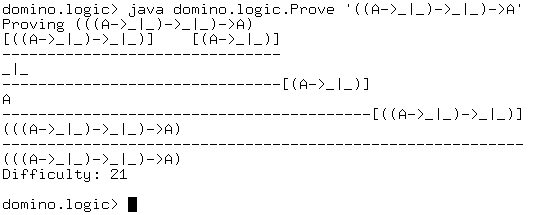## 4 Conclusion

Although the domino game in its present state is limited to propositional logic, it permits some interesting observations regarding the general feasibility and effectiveness of using a purely graphical system for constructing proofs.

One evident advantage is the compactness of presentation that a graphical system can offer. When you compare a domino tree with its corresponding proof in traditional notation, the domino tree is usually much smaller. In addition to this, the patterns and colors used by the domino game give much better visual clues than the corresponding textual formulae, especially when they are long and complex.

Another advantage has already been hinted at in the introduction by Peirce's statement that "all knowledge without exception comes from observation". An abstract graphical system offers a lot of room for playing around and observing patterns, something that is difficult with traditional textual systems because they are burdened with semantics that lead the mind into a certain direction.

Finally, a positive aspect that must not be overlooked is the fact that the domino game offers a more interesting experience than textual interfaces. This can improve a user's motivation and endurance when working on a difficult problem.

A purely graphical proving system does not only have advantages. One serious disadvantage of purely graphical reasoning systems is the fact that much of the background knowledge a user may have about logic does not translate easily to the graphical system. A lot of things have to be relearned. One example concerning the domino metaphor is the recognition of trivial truths such as AA. The ability to spot these trivial tautologies in textual notation does not automatically result in the ability to spot the corresponding color patterns when working with the domino game.

Another disadvantage is that an abstract graphical system is a step further away from the problem domain. Results achieved through manipulations in the abstract system do not usually have a straightforward reading with regards to the problem being solved. A translation step is required. The same is true in the other direction. Facts about the problem domain can not be used without being first translated into an abstract presentation. As a consequence it is difficult to make use of semantic background information that does not fit into the formal framework.

Despite the disadvantages, the results show that the usefulness of graphical concepts is not limited to teaching tools, but that it can actually improve the effectiveness of an interactive reasoning system's user interface. More research in this area is certainly required, especially concerning ways to combine the traditional formula-based approach with diagrammatic reasoning to get the best of both worlds.

But graphical reasoning systems are not only interesting tools for logicians. They could be used to explore aspects of the human brain regarding logical thinking. It could be interesting to examine the way the domino program is used by different people, both with and without background knowledge about logic. PET scans could be used to determine if using the domino program causes activity in the same areas of the brain as reasoning with the conventional formula-based notation, or if the same result (a proof of a tautology) is achieved in a different way.

Because the game does not require the ability to read or write or in fact any language skills whatsoever, it could also provide a suitable platform for studies involving children, illiterate people or persons with mental disabilities. Studies using the domino game could provide new insights about how logical thinking works in the brain, especially in the absence of language.